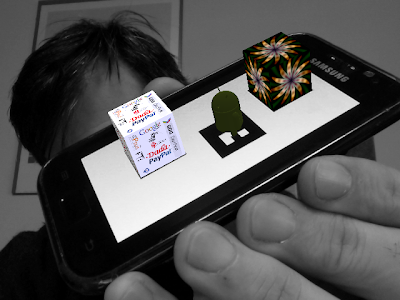## 21/11/12

### GWT Augmented Reality - HOWTO - step 2

Welcome to the third (and fortunately last) step of using NyARToolkit in a GWT project.

In the previous steps (step 0 and step 1) we got NyARToolkit ready for our GWT project and we
used  the toolkit to detect a marker on a static image; now we are going use the stream from the webcam to populate the sensor.

First of all we should now really switch to GWT 2.5 and Elemental; quoting form the first post of the series:

Using Elemental in a GWT project is quite straightforward:
1. donwload the latest GWT (well, we used for this project RC1 but all seems safe to be done with 2.5.0 final) and setup a new project;
2. add to the build path gwt-elemental.jar (is in the unpacked gwt 2.5 archive);
3. add to the gwt.xml file the line  <inherits name='elemental.Elemental'/>
....

Obtaining the stream from the camera

Create a video element:
final VideoElement videoElement =
Browser.getDocument().createVideoElement();

ask the browser for the webcam's stream:

bindVideoToUserMedia(videoElement, doneCallback);

where bindVideoToUserMedia is almost identical of the one published on https://github.com/henrikerola/FaceLogin/ whith added the DoneCallback we find useful.

public  void
bindVideoToUserMedia(final VideoElement video,
DoneCallback dc) {

final Mappable map = (Mappable) JsMappable.createObject();
map.setAt("video", true);

Browser.getWindow().getNavigator().webkitGetUserMedia(map,
new NavigatorUserMediaSuccessCallback() {
public boolean
onNavigatorUserMediaSuccessCallback(
LocalMediaStream stream) {
// video.setSrc(stream);
setVideoSrc(video, stream);
video.play();

dc.done();
return true;
}

}, new NavigatorUserMediaErrorCallback() {
@Override
public boolean
onNavigatorUserMediaErrorCallback(
NavigatorUserMediaError error) {
return false;
}
});
}

Once populated the video tag with the stream coming from the webcam we should  write the video into a canvas in order to be able to process the data.

Writing the video into a 2D-Canvas is straightforward (this is a buffer we use to get ImageData, not the final canvas where we will "augment" the video so the power of WebGL is not necessary here)

ctx.drawImage(videoElement, 0, 0);

as well as getting the image data,

ImageData imageData = ctx.getImageData(0, 0, w, h);

a simple "native cast" then give us the result as a byte[]

byte[] bytes = toArrayOfBytes(imageData.getData());

private static native byte[]
toArrayOfBytes(Uint8ClampedArray a) /*-{
return a;

}-*/;

that we should finally make a copy:

for(int j=0; j< len; j++) {
buffer[4*j]   = bytes[4*j+2];    //b
buffer[4*j+1] = bytes[4*j+1];  //g
buffer[4*j+2] = bytes[4*j];      //r
buffer[4*j+3] = bytes[4*j+3];  //a
}

We can now use the  NyARRgbRaster class to let NyARToolit  use our frame

i_input = new NyARRgbRaster(w, h,
NyARBufferType.BYTE1D_B8G8R8X8_32,false);
i_input.wrapBuffer(raster);
i_sensor.update(i_input);
nyar.update(i_sensor);

and finally we can ask nyar if our marker (added to the markersystem in the previous post) is detected:

boolean found = nyar.isExistMarker(marker_id);

in case the marker has been detected we can get the transformation matrix of the marker:

NyARDoubleMatrix44 mm = nyar.getMarkerMatrix(marker_id);

Having the matrix let us place any 3Dobject in the exact position of the detected marker.

To produce the "augmented" video steps are now quite clear:

1. prepare a canvas and get a  WebGLRenderingContext from it (see e. g. http://jooink.blogspot.it/2012/09/gwt-elemental-webgl-fundamentals.html)
2. write the video as a texture of a "far" rectangle occupying the entire viewport (the same post where ImageElement changet into VideoElement)
3. setup a 3D scene (here a good starting point)
4. remember to use the same projection matrix of the markersystem simply:

toCameraFrustumRH(i_config.getNyARParam(),1,0.1,1000.0,
perspectiveMatrix);

public static void toCameraFrustumRH(NyARParam i_arparam,
double i_scale,double i_near,double i_far,

double[] o_gl_projection) {
toCameraFrustumRH(
i_arparam.getPerspectiveProjectionMatrix(),

i_arparam.getScreenSize(),
i_scale,i_near,i_far,o_gl_projection);
return;
}
public static void toCameraFrustumRH(

NyARPerspectiveProjectionMatrix i_promat,
NyARIntSize i_size,double i_scale,double i_near,

double i_far,double[] o_gl_projection) {
NyARDoubleMatrix44 m=new NyARDoubleMatrix44();

i_promat.makeCameraFrustumRH(i_size.w,i_size.h,
i_near*i_scale,i_far*i_scale,m);
m.getValueT(o_gl_projection);
return;
}

5. transform the MarkerMatrix to the right space before using it, e.g.:

void toCameraViewRH(NyARDoubleMatrix44 mat,double i_scale, double[] o_gl_result)
{
o_gl_result[0 + 0 * 4] = mat.m00;       o_gl_result[1 + 0 * 4] = -mat.m10;
o_gl_result[2 + 0 * 4] = -mat.m20;          o_gl_result[3 + 0 * 4] = 0.0;
o_gl_result[0 + 1 * 4] = mat.m01;        o_gl_result[1 + 1 * 4] = -mat.m11;
o_gl_result[2 + 1 * 4] = -mat.m21;        o_gl_result[3 + 1 * 4] = 0.0;
o_gl_result[0 + 2 * 4] = mat.m02;        o_gl_result[1 + 2 * 4] = -mat.m12;
o_gl_result[2 + 2 * 4] = -mat.m22;        o_gl_result[3 + 2 * 4] = 0.0;

double scale=1.0/i_scale;
o_gl_result[0 + 3 * 4] = mat.m03*scale;

o_gl_result[1 + 3 * 4] = -mat.m13*scale;
o_gl_result[2 + 3 * 4] = -mat.m23*scale;

o_gl_result[3 + 3 * 4] = 1.0;
return;
}

That's definitely all :)DEMO

Ciao,
Alberto